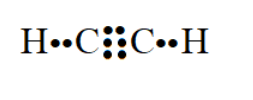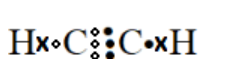# Draw electron dot structure of ethyne.Verified
139.7k+ views
Hint: The molecular formula of ethyne is ${{\text{C}}_2}{{\text{H}}_2}$. It contains a triple bond between two carbon atoms.
It also contains two carbon hydrogen single bonds. The number of valence electrons of carbon and hydrogen atoms are 4 and 1 respectively.

Ethyne is also known as acetylene. It is an unsaturated hydrocarbon containing a multiple bond. It contains two elements carbon and hydrogen. The elemental symbols for carbon and hydrogen are C and H respectively. In one molecule of ethyne, two carbon atoms and two hydrogen atoms are present. The molecular formula of ethyne is ${{\text{C}}_2}{{\text{H}}_2}$.
Each carbon atom has four valence electrons whereas each hydrogen atom has only one valence electron. A carbon atom can form four bonds whereas a hydrogen atom can form only one bond.

Calculate total number of valence electrons in ethyne molecules.

$2\left( 4 \right) + 2\left( 1 \right) = 8 + 2 = 10$
A covalent bond is formed when two electrons are shared. If 10 electrons are present, then, $\dfrac{{10}}{2} = 5$ bonds will be present.

To write the electron dot structure, put two hydrogen atoms at terminal positions and two carbon atoms in the internal positions. All the four atoms are placed in one line.

${\text{HCCH}}$

Now place one covalent bond between two carbon atoms and place two covalent bonds between carbon and hydrogen atoms.

${\text{H}} - {\text{C}} - {\text{C}} - {\text{H}}$

Out of 5 bonds, three bonds are accounted for and two bonds are remaining. Place these two bonds between two carbon atoms.

${\text{H}} - {\text{C}} \equiv {\text{C}} - {\text{H}}$

This is the Lewis dot structure. It can also be written as follows:It can also be written as follows:Note:
In the correct electron dot structure, each carbon atom has completed its octet whereas each hydrogen atom is surrounded by two electrons. Since each carbon atom forms four bonds, it is surrounded by eight electrons. Since each hydrogen atom forms one bond, it is surrounded by two electrons. Each bond represents a pair of electrons. In a compound, a hydrogen atom attains stability when it is surrounded by two electrons. In a compound, all atoms except the hydrogen atom attain stability when they are surrounded by eight electrons.